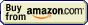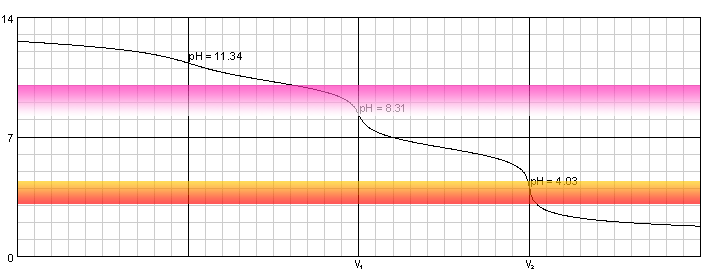# Acid-base titration## Acid-base titration » Warder titration

Balancer and stoichiometry calculator

operating systems:
XP, Vista, 7, 8, 10

€24.95 - approximately \$33

By clicking Buy Now! you will continue to the FastSpring checkout page where payment will be taken, and your order fulfilled by FastSpring, our trusted reseller, and Merchant of Record.

30-day money back guarantee!

### general remarks

Sodium hydroxide - both solid and dissolved - easily reacts with atmospheric carbon dioxide. That means it is usually contaminated with disodium carbonate Na2CO3. It is not a problem to determine sum of hydroxide and carbonates concentration by titration with a strong acid (although presence of dioxide means end point detection can be a little bit tricky). However, quite often we can be interested in the amount of carbon dioxide absorbed. We can try to determine amount of carbonates present in the solution by gravimetric method (precipitation of barium carbonate). However, it is possible to determine both sodium hydroxide and carbonate in one titration. Results will be not as precise as in the case of gravimetric method, nonetheless method is very fast and simple and often accurate enough.

How is it possible? Let's take a look at the titration curve of mixture containing 0.1 M of sodium hydroxide and 0.1 M of disodium carbonate titrated with hydrochloric acid.Warder titration - solution of 0.1M NaOH and 0.1M Na2CO3 titrated with 0.1M solution of strong acid. Carbonic acid dissociation constants: pKa1=6.37, pKa2=10.25.

Solution contains three bases - OH-, CO32- and HCO3-. Differences between their strengths are large enough so that there are three inflection points on the titration curve. First one - at pH 11.3 - is barely visible and for sure can't be detected using pH indicators. Second and third are much better. Even if the steep parts of the curve are relatively short, they happen in the areas where two most popular indicators (phenolphthalein and methyl orange) change their colors. Also, in low pH phenolphthalein is colorless, which makes detection of methyl orange color change easier. Question is - what is titrated at these end points?

Obviously, the stronger the base, the easier it reacts with acid. Of the three bases present, NaOH is the strongest, so it will be neutralized first. Next one is CO32- - as pKa2=10.25, pKb1=3.75 (see polyprotic dissociation constants for Ka/Kb conversion details). When all CO32- is converted to HCO3-, pH of the solution is approximately (pKa1+pKa2)/2=(6.37+10.25)/2=8.31 (see pH of amphiprotic substance solution). pH at which phenolphthalein starts or ceases to be visible is 8.2, so if we titrate solution till color disappears, we can be sure we are left with solution of HCO3-. That means after adding V1 mL of the titrant we have titrated sum of sodium hydroxide and disodium carbonate:

NaOH + HCl → NaCl + H2O

Na2CO3 + HCl → NaCl + NaHCO3

Solution contains now only one base - HCO3-. Once it gets protonated, we will be left with solution of carbonic acid, with pH around 4.0. This is close to the pH at which methyl orange starts to change color (when approached from the high pH side), which is usually listed as 4.4. Reaction that took place during this second stage of titration was

NaHCO3 + HCl → NaCl + H2O + CO2

and obviously amount of titrant added (V2-V1) reflects only amount of carbonate. That in turn means that to calculate amount of sodium hydroxide we should use V1-(V2-V1) = 2V1-V2 as a titrant volume.

Warder method gives best results when the amount of sodium carbonate is substantially lower than the amount of sodium hydroxide.

To avoid loss of carbon dioxide solution should be kept cold during titration and not shaken.

### reaction

There are three reactions taking place during titration:

NaOH + HCl → NaCl + H2O

Na2CO3 + HCl → NaCl + NaHCO3

NaHCO3 + HCl → NaCl + H2O + CO2

or two overall reactions:

NaOH + HCl → NaCl + H2O

and

Na2CO3 + 2HCl → 2NaCl + H2O + CO2

### sample size

When using 0.2 M hydrochloric acid, optimal amount of sodium hydroxide in an aliquot is 0.20-0.25 g and of carbonate - 0.04-0.07 g.

### end point detection

As discussed in general remarks section, we detect two end points - first one using phenolphthalein, second one using methyl orange. In the first case we titrate till solution loses pink color, in the second case - to the first color change.

### solutions used

To perform titration we will need titrant - 0.2 M or 0.1 M hydrochloric acid solution, indicators - phenolphthalein & methyl orange, and some amount of distilled water to dilute sample.

### procedure

• Pipette aliquot of sodium hydroxide and sodium carbonate solution into 250mL Erlenmeyer flask.
• Dilute with distilled water to about 100 mL.
• Add 2-3 drops of phenolphthalein solution.
• Cool it down in an ice bath.
• Titrate with HCl solution till the color disappears. Write down first end point volume V1.
• Add 1-2 drops of methyl orange solution.
• Titrate with HCl solution till the first color change.

To avoid loss of carbon dioxide it is good to avoid vigorous mixing. If possible, we should keep end of the burette submersed in the titrated solution.

### result calculation

As it was explained above, result of the titration requires use of two volumes - first end point volume V1 and second end point volume V2. Amount of sodium hydroxide can be calculated using stoichiometry of the reaction

HCl + NaOH → NaCl + H2O

and 2V1-V2 volume as titrant volume, while amount of carbonate can be calculated using stoichiometry of the reaction

Na2CO3 + HCl → NaCl + NaHCO3

and V2-V1 as a titrant volume. In other words in both cases we can assume 1:1 stoichiometry.

To calculate sodium hydroxide solution concentration use EBAS - stoichiometry calculator. Download determination of sodium hydroxide concentration reaction file, open it with the free trial version of the stoichiometry calculator.

Click button above HCl in the input frame, enter 2V1-V2 and concentration of the titrant used. Click button. Read number of moles and mass of sodium hydroxide in the titrated sample in the output frame. Click button in the output frame below sodium hydroxide, enter volume of the pipetted sample, read sodium hydroxide concentration.

To calculate sodium carbonate solution concentration use EBAS - stoichiometry calculator. Download determination of sodium carbonate concentration reaction file, open it with the free trial version of the stoichiometry calculator.

Click button above HCl in the input frame, enter V2-V1 and concentration of the titrant used. Click button. Read number of moles and mass of sodium carbonate in the titrated sample in the output frame. Click button in the output frame below sodium carbonate, enter volume of the pipetted sample, read sodium carbonate concentration.

### sources of errors

Warder titration is not an easy one. First of all, both end points are not very well visible (curve is not too steep), so accurate judgement of color is very important. Second, during titration (especially before first end point) it is possible to lose some carbon dioxide from the solution. If that happens we will overestimate amount of sodium hydroxide and underestimate amount of carbonate. Other important point is that both calculations of amount of carbonate and hydroxide are based on two measurements, which means final error can be twice as large as in the case of simple titration with one end point.

Many variants of Warder titration have been proposed to minimalize these problems. Some call for the titration in closed vessels, where carbon dioxide can't run away, others call for diluted titrant used to reach second end point and so on.

Then there are all possible general sources of titration errors.